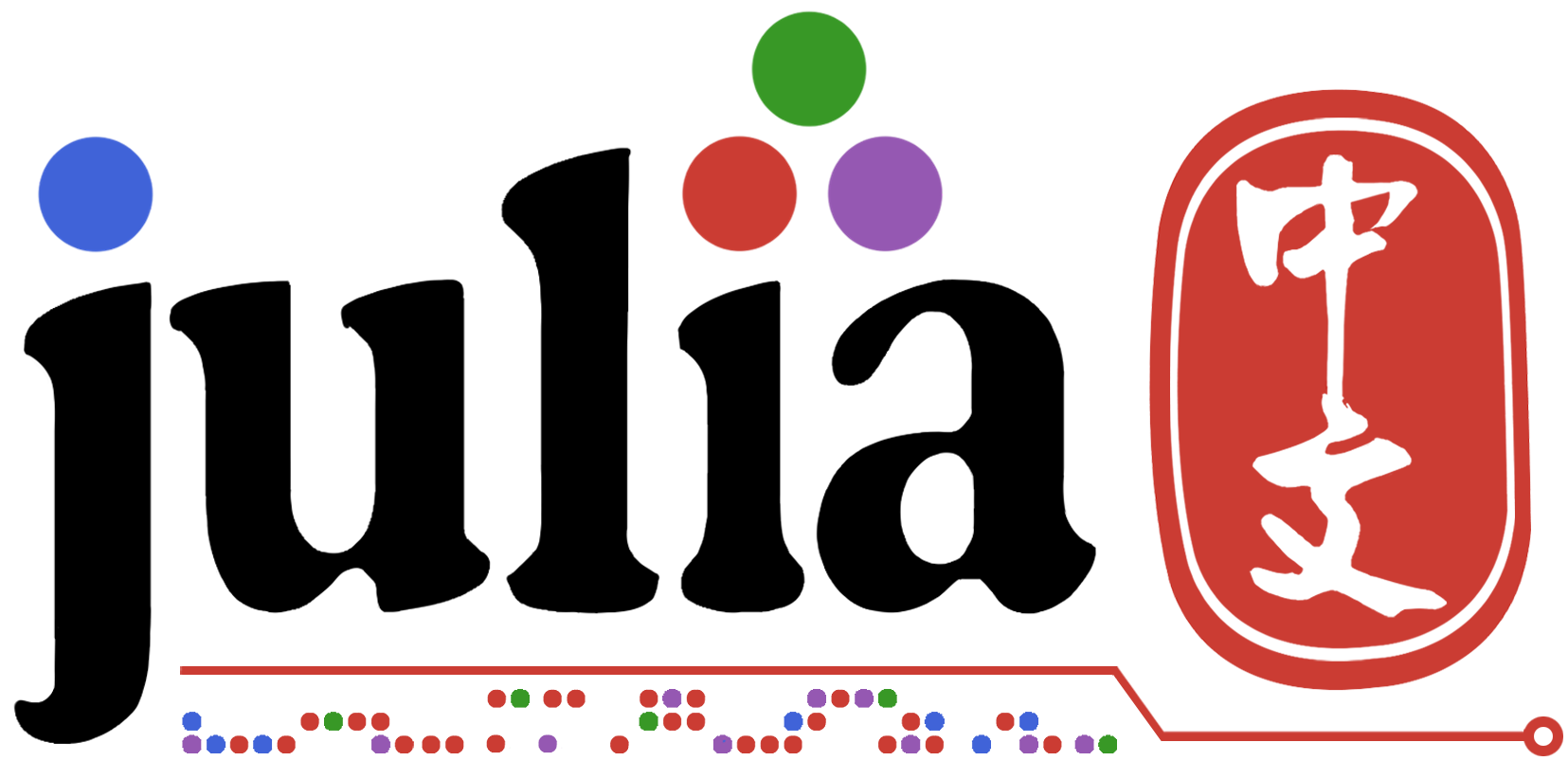# 请问julia里 Dictionary 如何进行 update

`dict.update({k: v[t,...] for k, v in kwargs.items()})`

`merge`方法

1赞

``````d = Dict(zip(['a', 'b', 'c'], 1:3))
d['a'] = 10
``````

1赞

``````merge!(dict, Dict(k=>v[t,...] for (k,v) in kwargs))
``````
1赞